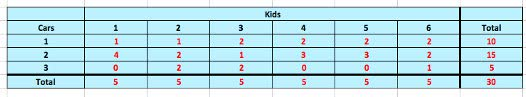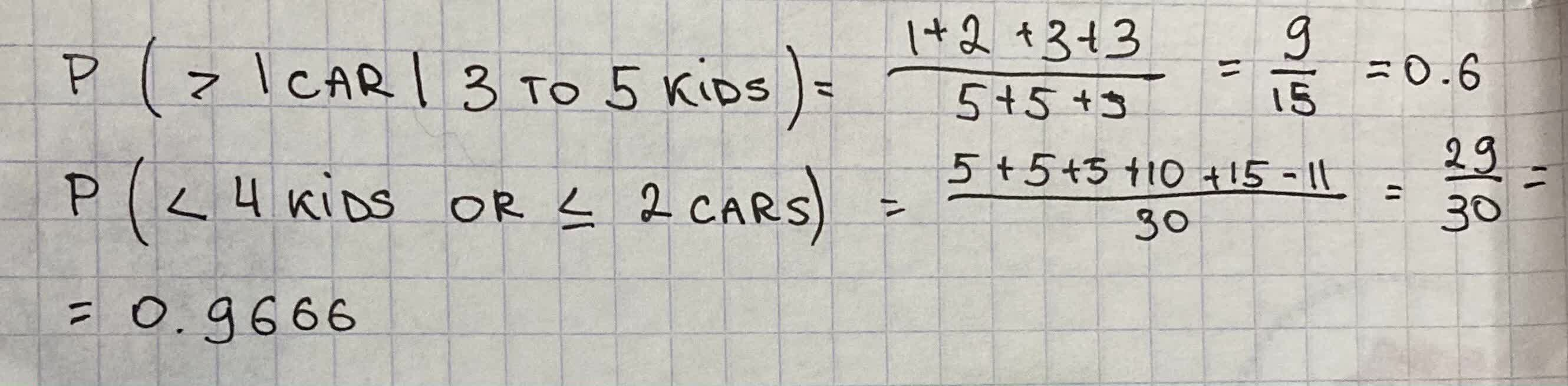Answer the question using the table.1) P (> 1 car | 3 to 5 kids) = ? 2)P (< 4 kids or <= 2 cars) = ?Cem Hayes 2021-09-07 Answered
Answer the question using the table.1) P (> 1 car | 3 to 5 kids) = ?
2)P (< 4 kids or $$\displaystyle\le$$ 2 cars) = ?

Want to know more about Two-way tables?

• Questions are typically answered in as fast as 30 minutes

Solve your problem for the price of one coffee

• Math expert for every subject
• Pay only if we can solve itSabadisO

Calculation: